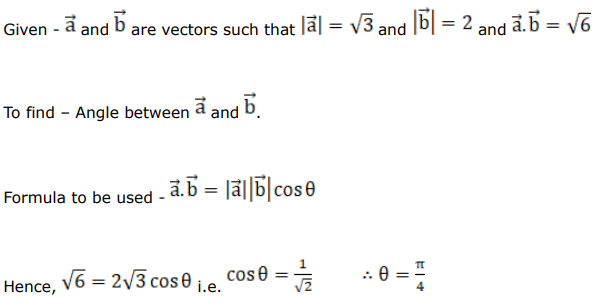# Mark against the correct answer in each of the following:

Question:

Mark $(\sqrt{)}$ against the correct answer in each of the following:

f $\vec{a}$ and $\vec{b}$ are vectors such that $|\vec{a}|=\sqrt{3},|\vec{b}|=2$ and $\vec{a} \cdot \vec{b}=\sqrt{6}$ then the angle between $\vec{a}$

and $\overrightarrow{\mathrm{b}}$ is

A. $\frac{\pi}{6}$

B. $\frac{\pi}{3}$

C. $\frac{\pi}{4}$

D. $\frac{2 \pi}{3}$

Solution: